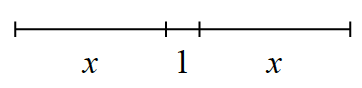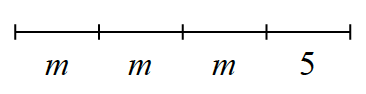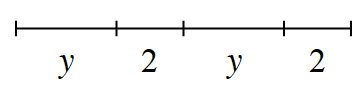### Home > CC1 > Chapter 4 > Lesson 4.2.4 > Problem4-82

4-82.

Use an algebraic expression to represent each sequence of lengths shown below.

1.Think of this as being a tightrope for Croakie to cross. How would you describe his path using this picture?

$2x + 1 \text{ or } x + 1 + x$

1.This problem is very similar to part (a).
If the lengths labeled ''$m$'' were instead labeled as ''$x$'', how would you write the expression?

$3m + 5 \text{ or } m + m + m + 5$

1.Use parts (a) and (b) as guides if you are having trouble. Remember that the variable represents a number, so you can use it in an expression just as you would a number.